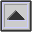ZERO LEVEL VU

A reference power level shown on a VU METER. Although 0 VU is shown on a VU meter as the point of 100% MODULATION, it is not identical to the reference called zero level, which in fact refers to -4 dB or -4 VU on the meter.

Zero level is the reference level obtained with a 1000 Hz signal and 1 milliwatt of power in a line or circuit of 600 ohms resistance (a 600-ohm line). The corresponding voltage level is 0.773 volts. This level is sometimes called 0 dbm. Other levels may be described in reference to it; for instance, 0 VU is +4 dbm and represents a voltage level of 1.228 volts.

Compare: DECIBEL, THRESHOLD OF HEARING.

home